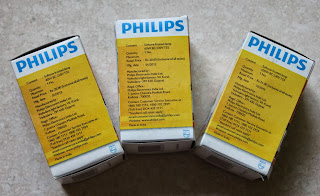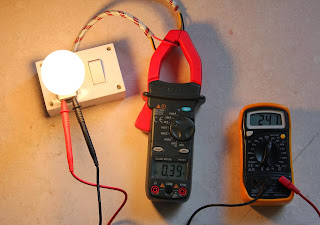## The Bulbs

So I took 3 bulbs of 40 Watts, 60 Watts and 100 Watts respectively and measured the hot and cold resistances

I used the Philips softtone frosted lamp series.

Price:
40 Watts : Rs 20
60 Watts : Rs 20
100 Watts  : Rs 21Blub packs - FrontBlub packs - Back

## Cold Resistances

The cold resistance readings were as follows:

40 Watts : around 97.6 Ohms
60 Watts : around 71.4 Ohms
100 Watts : around 39.5 OhmsMeasuring Cold resistance of 40W BulbMeasuring Cold resistance of 60W BulbMeasuring Cold resistance of 100W Bulb

## Hot Resistances (Calculated)

Calculated values of hot resistance can be obtained using the formula: Resistance = (Voltage^2) / Power
In India the AC voltage RMS value is 230 Volts.

So we get the hot resistances as:

40W Bulb : (230*230) / 40 = 1322.5 Ohms
60W Bulb : (230*230) / 60 = 881.67 Ohms
100W Bulb : (230*230) / 100 = 529 Ohms

## Hot Resistances (Measured)

To get the actual values of the hot resistance, you have to measure the current flowing through the bulb. In case of AC supply, this can be done using a clamp meter. It measures the current flowing through a wire by sensing the amount of magnetic flux induced around it. This allows one to measure the current flowing through the wire without making physical contact with the wire conductors. The current should be measured only by clamping it around one of the two wires - live or neutral. Each of these wires carry current in opposite directions so if you clamp the meter around both the wires together, the current will read as zero because the magnetic fields induced in the clamp jaw will cancel each other out.

We can assume the AC mains voltage to be constant at 230Vrms but it does vary from place to palace, so I connected a multimeter in parallel with the bulb contacts to measure the voltage too.

The measured values are:

40W Bulb : 242 / 0.15 = 1613.33 Ohms
60W Bulb : 241 / 0.23 = 1052.17 Ohms
100W Bulb : 241 / 0.39 = 617.95 OhmsMeasuring the hot resistance of a 40W BulbMeasuring the hot resistance of a 60W BulbMeasuring the hot resistance of a 100W Bulb

## Measuring the power directly using a digital energy meter

Now days small digital energy meters are available which can measure power consumed by a single device during a period of time. In India, you can get one from Visha Electronics, Mumbai (http://www.vishaworld.com/). I just wanted to check by how many watts did the value of the actual power consumed vary from the one printed on the box.

40W Bulb : 42.9 Watts
60W Bulb : 64.1 Watts
100W Bulb : 104 WattsMeasuring the power consumed by a 40W Bulb using a digital energy meterMeasuring the power consumed by a 60W Bulb using a digital energy meterMeasuring the power consumed by a 100W Bulb using a digital energy meter

## Insides of a digital energy meter

1.Awsome! Great post, very clear and thorough. Makes understanding much simpler without the heavy duty mathematical equations. What would have been nice would be a graph showing the wattage, voltage, current, and resistance values migrate up, or down. Maybe I'll try to tackle that after your great enlightenment post. Thank you very much from San diego California USA.

2.Whats the difference between hot and cold resistance?

3.Good to know, I was trying to work out a bulb wattage by measuring the resistance of the filament, and was confused why the reading was 50ohm cold reading on a 250 volt supply that would have been almost 1kw, that's impossible for a G9 bulb as they only make them up to 60 watts. My sanity is now restored after reading this useful information. Thank you.

4.i was myself confused while trying to measure the inductance of a coil in series with a bulb by measuring the potential drop across the bulbs and the inductor... the resistivity of the element of bulb changes with temperature at a very high rate ... it has low resistivity on low temperatues.. this is so that even at low voltages power consumed Vsqr/R remains high and we get a more glowing effect even at low voltages

5.really helpful. thank you ! <3